# 27? 73 S2 0.20A 0.001Fv(t) i(t) -10t The capacitor voltage in this circuit is v(t)- 14.60-33.6...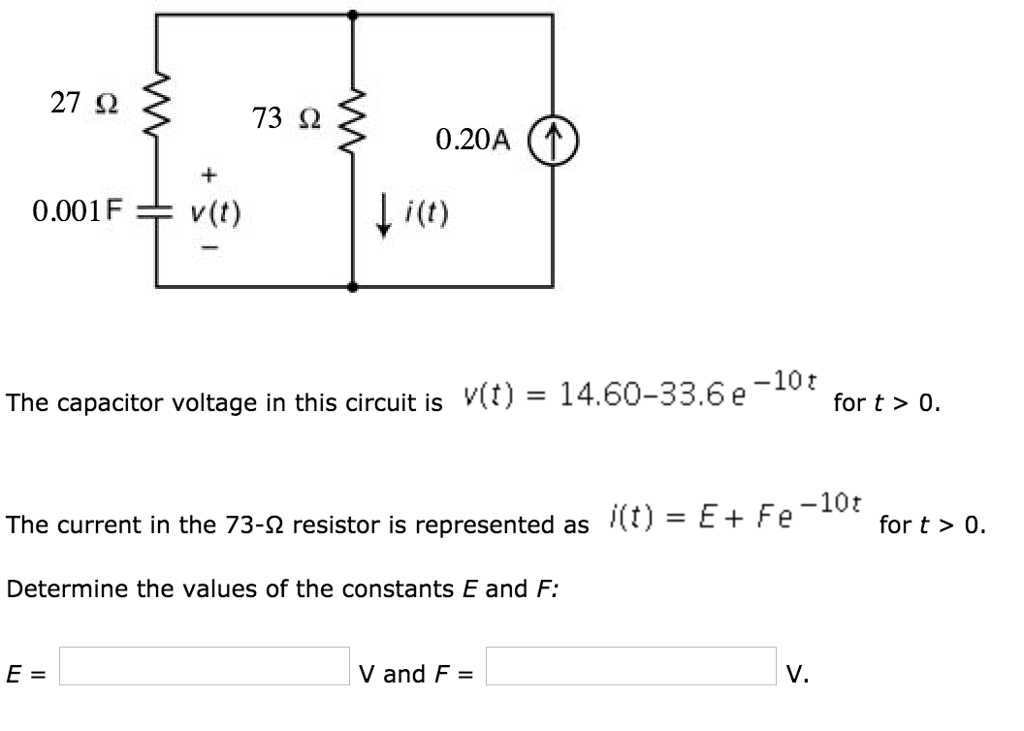27? 73 S2 0.20A 0.001Fv(t) i(t) -10t The capacitor voltage in this circuit is v(t)- 14.60-33.6 efort 0. -10t The current in the 73-? resistor is represented as ,(t)-E+ Fe - for t > 0. Determine the values of the constants E and F: V and F V.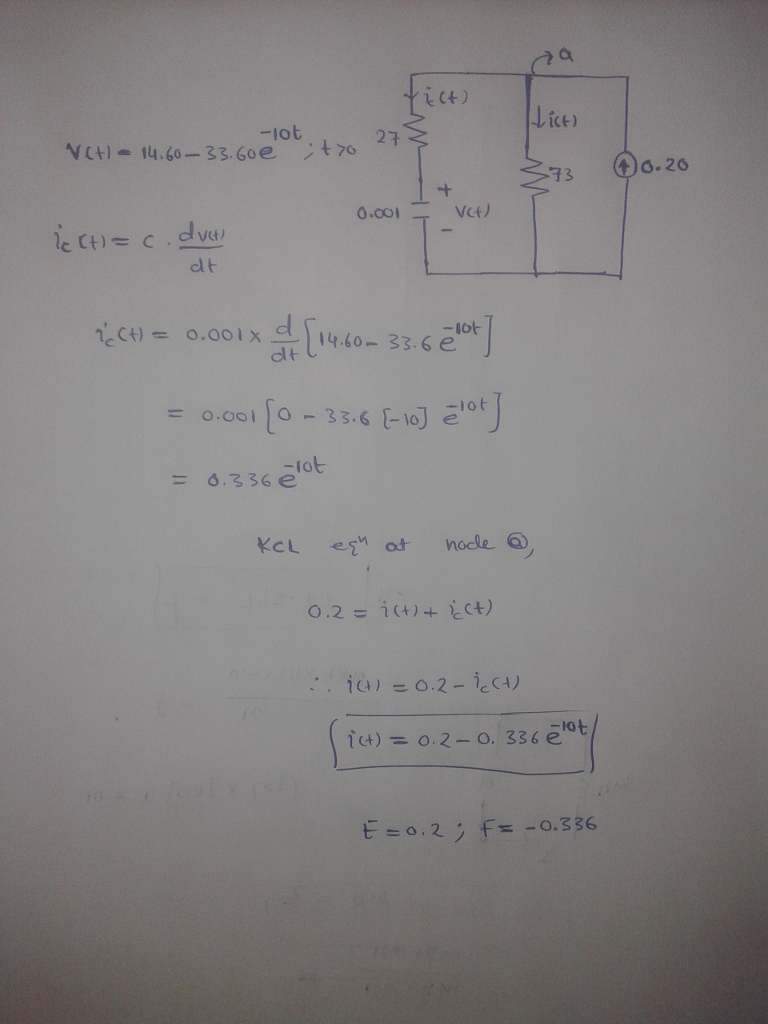##### Add Answer of: 27? 73 S2 0.20A 0.001Fv(t) i(t) -10t The capacitor voltage in this circuit is v(t)- 14.60-33.6...
Similar Homework Help Questions
• ### Problem 10.10-1 72 kS2 The input to this circuit is the voltage source voltage given by...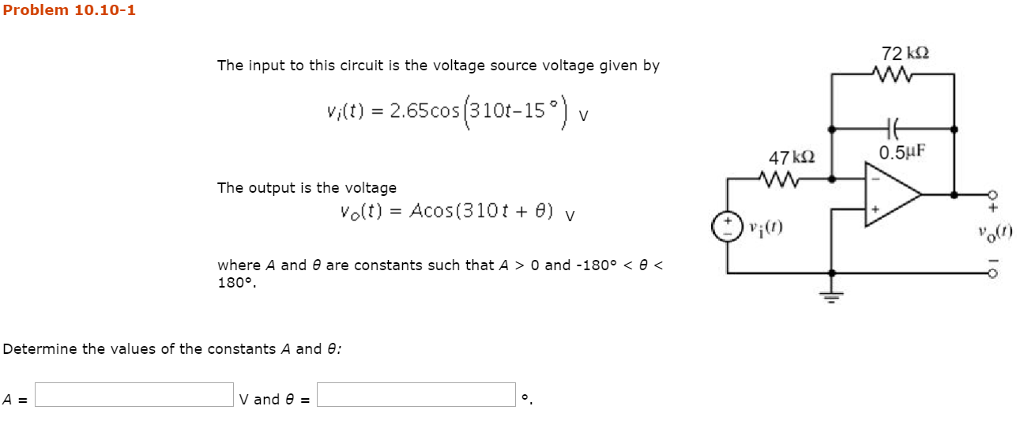Problem 10.10-1 72 kS2 The input to this circuit is the voltage source voltage given by v(t) 2.65cos 310t-15° v 0.5HF The output is the voltage Vo(t)-Acos(310 t + ?) v vi0) ou) where A and ? are constants such that A > 0 and-180° < ? < 180°. Determine the values of the constants A and ?: | V and ?

• ### 3. (25 pts) The circuit is at steady state before the switch closes. Determine the capacitor...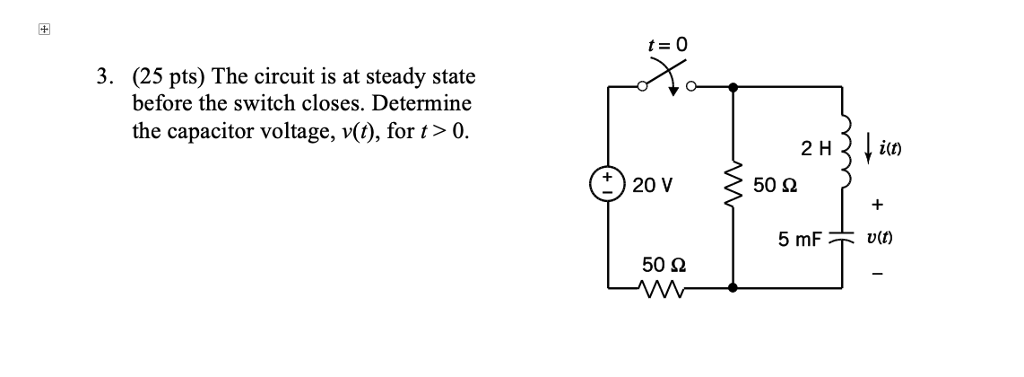3. (25 pts) The circuit is at steady state before the switch closes. Determine the capacitor voltage, v(), for t> 0 2 Hin + )20 V 50 2 50 2

• ### The voltage of the capacitor vo(t) for t> 0 is known to be vo(t) = 9e-20...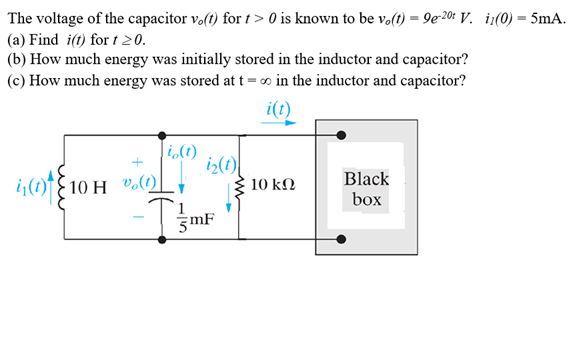The voltage of the capacitor vo(t) for t> 0 is known to be vo(t) = 9e-20 V. i1(0) = 5mA. (a) Find i(t) for t 20. (b) How much energy was initially stored in the inductor and capacitor? (c) How much energy was stored at t= in the inductor and capacitor? it) (0) i20 1110 H V.0) 10 kΩ Black box -T gmF

• ### I need help with these Laplace problems:) (1 point) Find the Laplace transform of <9 f(t)...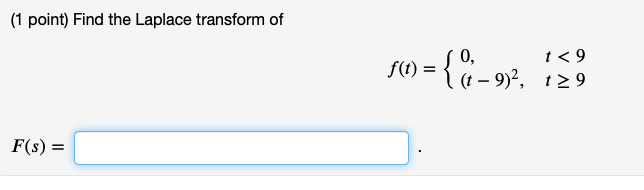I need help with these Laplace problems:) (1 point) Find the Laplace transform of <9 f(t) = { 0, " I(t - 9)?, 129 F(s) = (1 point) Find the inverse Laplace transform of e-75 F(s) = 52 – 2s – 15 f(t) = . (Use step(t-c) for uc(t).) (1 point) Find the Laplace transform of 0. f(t) t<5 112 – 10t + 30, 125 F(s) =

• ### Problem 7.2-10 is(t), A 7.8 88 2 0.40F v(t) 3.9 t, S 0.50 1.00 Consider this circuit together wit...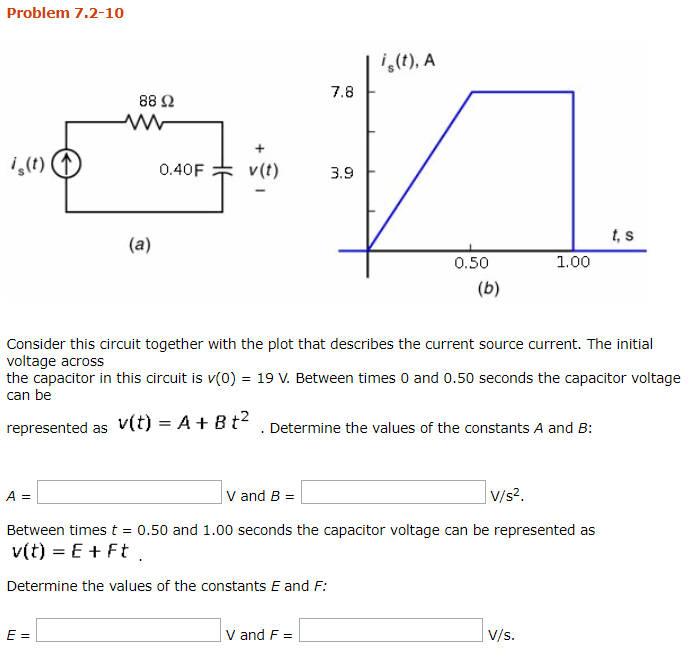Circuits Chapter #7 Problem 7.2-10 is(t), A 7.8 88 2 0.40F v(t) 3.9 t, S 0.50 1.00 Consider this circuit together with the plot that describes the current source current. The initial voltage across the capacitor in this circuit is v(o) 19 V. Between times O and 0.50 seconds the capacitor voltage can be represented as v(t) = A + B t2 . Determine the values of the constants A and B: V/s2 A= Between times t 0.50 and 1.00...

• ### Problem 8 In the given circuit i(0) = 3A Determine: a) i(t) (5 points) and b)...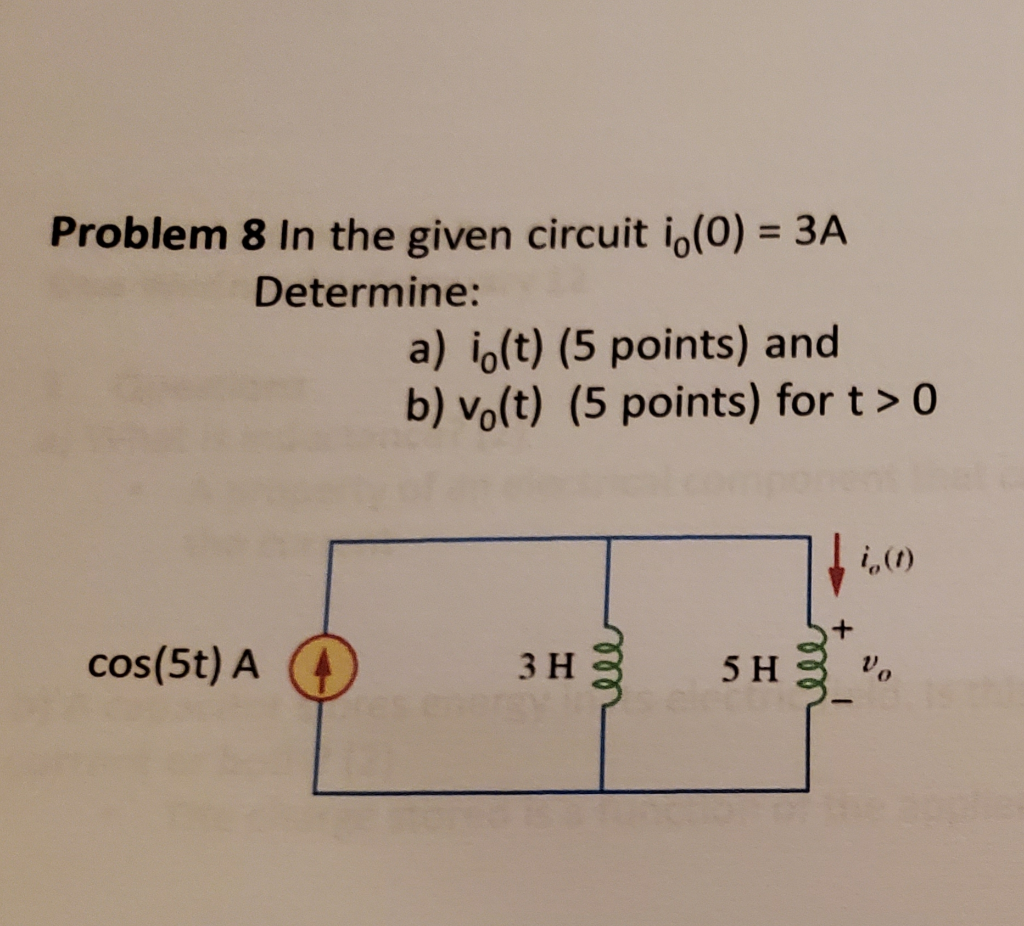Problem 8 In the given circuit i(0) = 3A Determine: a) i(t) (5 points) and b) vo(t) (5 points) for t > 0 cos(5t) A 0 3h 5 *,

• ### 8.5 Find i for t> 0 if (0) 4 V. 6Ω 2i V 3Ω PROBLEM 8.5...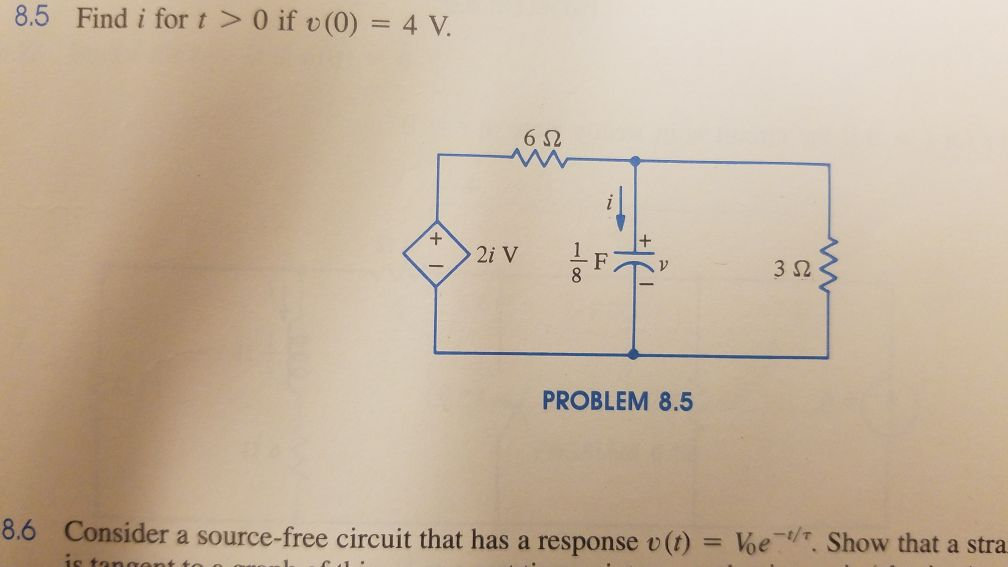8.5 Find i for t> 0 if (0) 4 V. 6Ω 2i V 3Ω PROBLEM 8.5 8.6 Consider a source-free circuit that has a response v(t) Voe. Show that a stra

• ### Question 4) In the circuit in (Figure 1) the voltage and current expressions are v=36e-25 V,...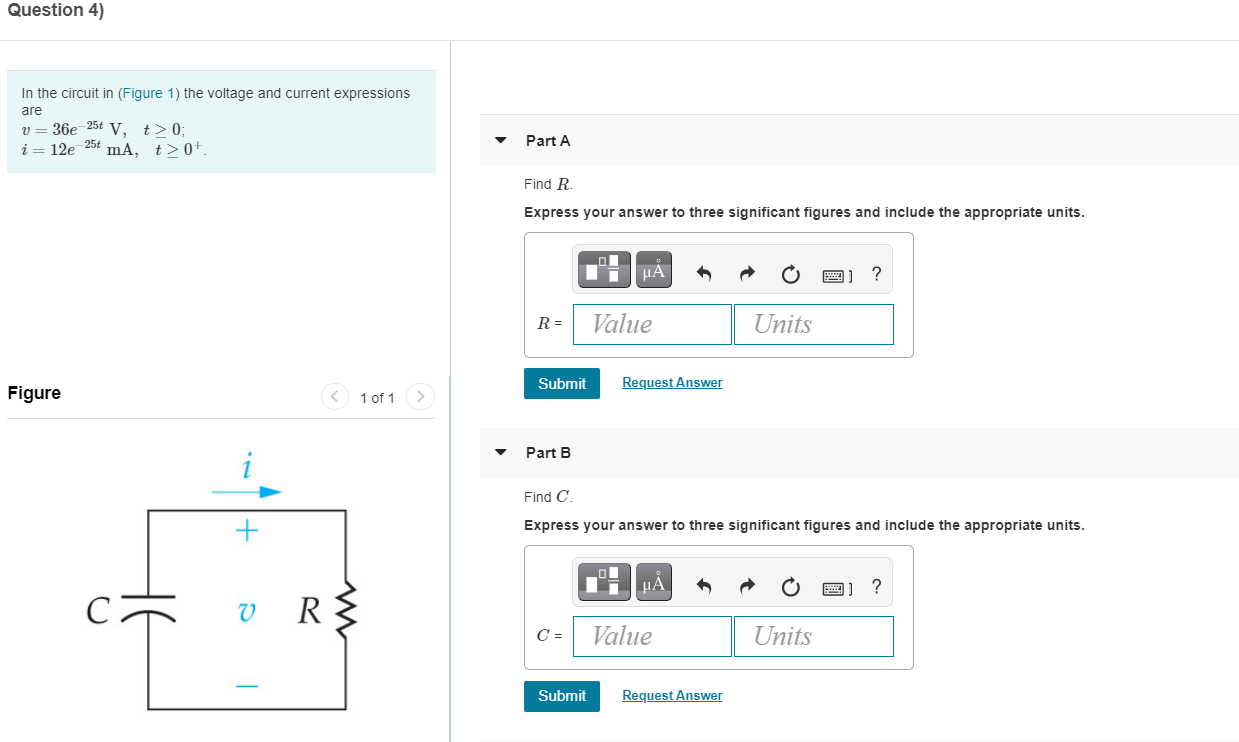Question 4) In the circuit in (Figure 1) the voltage and current expressions are v=36e-25 V, t>0; i= 12e-25 mA, +0+ Part A Find R Express your answer to three significant figures and include the appropriate units. μΑ ? R= Value Units Submit Request Answer Figure < 1 of 1 > Part B Find C + Express your answer to three significant figures and include the appropriate units. uA ? v RS C= Value Units Submit Request Answer Question 4)...

• ### In the circuit in (Figure 1) the voltage and current expressions are 60e -25t V, t...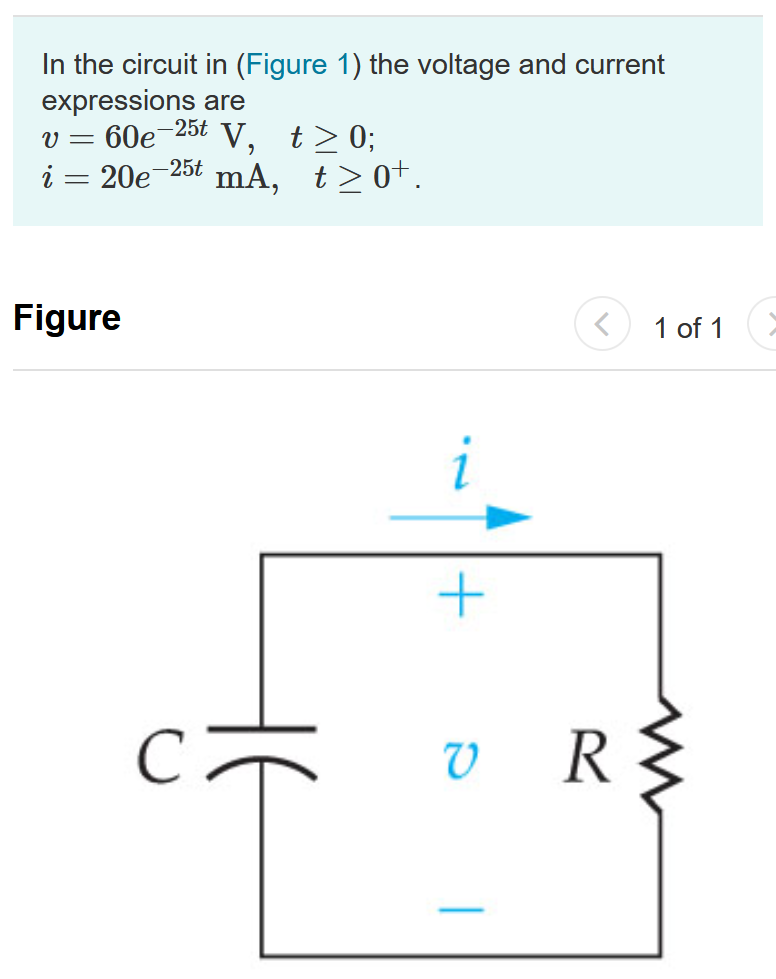In the circuit in (Figure 1) the voltage and current expressions are 60e -25t V, t > 0); i 20e -25t mA, t> 0+. V = Figure 1 of 1 + V R RS Part A Find R. Express your answer to three significant figures and include the appropriate units. ? MÅ R = Value Units Submit Request Answer Part B Find C. Express your answer to three significant figures and include the appropriate units. ? MÅ C = Value...

• ### In the circuit below, the voltage supplied by the power source is 10 V and the...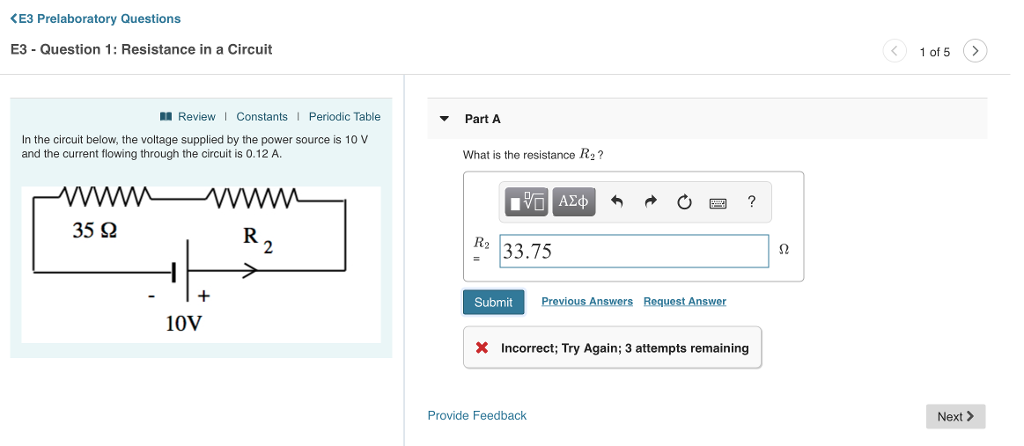In the circuit below, the voltage supplied by the power source is 10 V and the current flowing through the circuit is 0.12 A. KE3 Prelaboratory Questions E3 - Question 1: Resistance in a Circuit 1 of 5 Review 1 Constants i Periodic Table Part A In the circuit below, the voltage supplied by the power source is 10 V and the current flowing through the circuit is 0.12 A What is the resistance R2? 35 S2 33.75 Submit Previous...

Free Homework App